Practice the problems of Math in Focus Grade 3 Workbook Answer Key Chapter 10 Practice 1 Addition to score better marks in the exam.

Question 1.
$7.30 +$2.00 = $__________ Answer:$7.30 + $2.00 =$9.30
Explanation:
Perform addition operation on above two numbers. Add $7.30 with$2.00 the sum is $9.30. Question 2.$37.20 + $0.45 =$__________
$37.20 +$0.45 = $37.65 Explanation: Perform addition operation on above two numbers. Add$37.20 with $0.45 the sum is$37.65.

Question 3.
$5.20 +$12.65 = $__________ Answer:$5.20 + $12.65 =$17.85
Explanation:
Perform addition operation on above two numbers. Add $5.20 with$12.65 the sum is $17.85. Question 4.$51.20 + $14.80 =$__________
$51.20 +$14.80 = $66.00 Explanation: Perform addition operation on above two numbers. Add$51.20 with $14.80 the sum is$66.00.

Question 5.
$0.95 +$9.35 = $__________ Answer:$0.95 + $9.35 =$10.30
Explanation:
Perform addition operation on above two numbers. Add $0.95 with$9.35 the sum is $10.30. Question 6.$4.35 + $64.85 =$__________
$4.35 +$64.85 = $69.20 Explanation: Perform addition operation on above two numbers. Add$4.35 with $64.85 the sum is$69.20.

Question 7.
$31.65 +$3.90 = $__________ Answer:$31.65 + $3.90 =$35.55
Explanation:
Perform addition operation on above two numbers. Add $31.65 with$3.90 the sum is $35.55. Question 8.$5.45 + $0.75 =$__________
$5.45 +$0.75 = $6.20 Explanation: Perform addition operation on above two numbers. Add$5.45 with $0.75 the sum is$6.20.

Find each missing amount.

Question 9.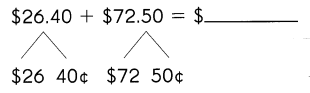$26 +$72 = $__________ 40¢ + 50¢ = __________¢$__________ + __________¢ = $__________ Answer: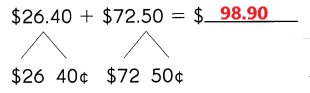$26 + $72 =$98
40¢ + 50¢ = 90¢
$98 + 90¢ =$98.90
Explanation:
In the above image we can observe before the decimal point the number is represented with $and after the decimal point the number is represented with ¢. The total sum is represented with$.
Add before the decimal point numbers.
$26 +$72 = $98 Add after the decimal point numbers. 40¢ + 50¢ = 90¢ Add before and after decimal point numbers.$98 + 90¢ = $98.90 Question 10.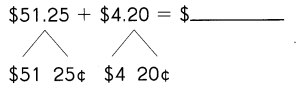$__________ + $__________ =$__________
__________¢ + __________¢ = __________¢
$__________ + __________¢ =$ __________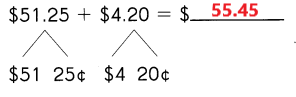$51 +$4= $55 25¢ +20¢ = 45¢$55 + 45¢ = $55.45 Explanation: In the above image we can observe before the decimal point the number is represented with$ and after the decimal point the number is represented with ¢. The total sum is represented with $. Add before the decimal point numbers.$51 + $4=$55
Add after the decimal point numbers.
25¢ +20¢ = 45¢
Add before and after decimal point numbers.
$55 + 45¢ =$ 55.45

Find each missing amount.

Question 11.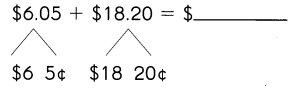$__________ +$__________ = $__________ __________¢ + __________¢ = __________¢$__________ + __________¢ = $__________ Answer: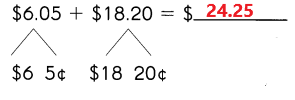$6 + $18 =$24
5¢ + 20¢ = 25¢
$24 + 25¢ =$ 24.25
Explanation:
In the above image we can observe before the decimal point the number is represented with $and after the decimal point the number is represented with ¢. The total sum is represented with$.
Add before the decimal point numbers.
$6 +$18 = $24 Add after the decimal point numbers. 5¢ + 20¢ = 25¢ Add before and after decimal point numbers.$24 + 25¢ = $24.25 Question 12.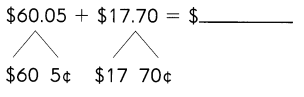$__________ + $__________ =$__________
__________¢ + __________¢ = __________¢
$__________ + __________¢ =$ __________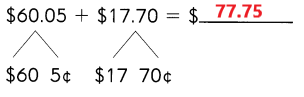$60 +$17 = $77 5¢ + 70¢ = 75¢$77 + 75¢ = $77.75 Explanation: In the above image we can observe before the decimal point the number is represented with$ and after the decimal point the number is represented with ¢. The total sum is represented with $. Add before the decimal point numbers.$60 + $17 =$77
Add after the decimal point numbers.
5¢ + 70¢ = 75¢
Add before and after decimal point numbers.
$77 + 75¢ =$ 77.75

Use the items and prices in the picture to answer each exercise.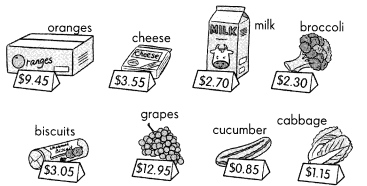What is the cost of
Question 13.
a carton of milk and a head of broccoli? $__________ Answer: A carton of milk cost is$2.70.
A head of broccoli cost is $2.30$2.70 + $2.30 =$ 5
The cost of both carton of milk and a head of broccoli are $5. Question 14. a bunch of grapes and a packet of whole meal biscuits?$__________
A bunch of grapes cost is $12.95. A packet of whole meal biscuits is$3.05.
$12.95 +$3.05 = $16 The cost of both bunch of grapes and a packet of whole meal biscuits are$16.
a cucumber and a head of cabbage? $__________ Answer: A cucumber cost is$0.85
A head of cabbage cost is $1.15.$0.85 + $1.15 =$2
The cost of both cucumber and a head of cabbage are $2. Question 16. a pack of cheese and a box of oranges?$__________
A pack of cheese cost is $3.55. A box of oranges cost is$9.45.
$3.55 +$9.45 = $13 The cost of both pack of cheese and a box of oranges are$13.